# 2. (a) Using table data presented in the text, calculate the focusing power Fmin of the crystallinelens in the case when eye is focused to view distant objects. (b) Knowing that focusing power of thecornea is Feornea = 42 D, calculate minimum focusing power of the eye. (c) Find what if the eyefocal length in this case. Eye optical system1. Optical power of the cornea:Fornea = F, + F =F- optical power of the cornea front surface, D; F- optical power of the cornea back surface, D; R,radius of curvature of the comea front surface, m;Clary fibersR.Vous humorComeaLene- radius of curvature of the comea back surface,R,Foram; n1 - ambient refraction index (for air n = 1 andfor water n, = 1.33); n - cornea refraction index:n- aqueous humour refraction index.2. Optical power of the erystalline lens:Ferystalline tens =F +F, = s-aE- optical power of the crystalline lens frontsurface, D; F, - optical power of the crystalline lens back surface, D; R - radius of curvature ofthe crystalline lens front surface, m; R, - radius of curvature of the crystalline lens back surface, m;n - aqueous humour refraction index; n, - crystalline lens refraction index; n; - vitreous humorOplo nerveAgueoushumorRenaScerarefraction index.Table - Parameters for the EyeRadius, mmIndex ofrefractionFrontBackR = 7.8Ramar =10Ramin =6Aqueous humour and vitreous humorR, = 7.3R4maz =6Ramie =5.5Cornean, =1.38n4 =1.4Crystalline lens (Fmin)Crystalline lens (Fmar)=1.333. Optical power of eye:F= Farnaa + Feristalline lens4. Lens equation:1 1. 1CorneaLensS- lens focal length (m); F- lens optical power (D); p- distance from the object to the lens or object distance(m); g – distance from the image to the lens or imagedistance (m).5. Height of image on the retina:h.9Н ЕH - height of image on the retina, m; h - height of object, m; q - image distance (for human eye onthe average is equal to 1.5 cm), m; p - distance of the object to optical system of the eye, m.6. Lens magnification:нH- image height (m); h - object height (m), p - object distance (m); g - image distance (m).7. Law of refraction:nisine, = nzsinez

Question
15 views

Can you use the formula in the second picture to answer it, please?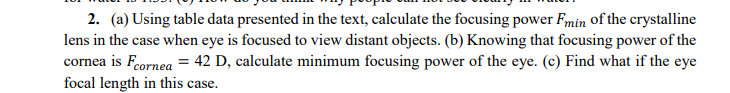help_outlineImage Transcriptionclose2. (a) Using table data presented in the text, calculate the focusing power Fmin of the crystalline lens in the case when eye is focused to view distant objects. (b) Knowing that focusing power of the cornea is Feornea = 42 D, calculate minimum focusing power of the eye. (c) Find what if the eye focal length in this case. fullscreen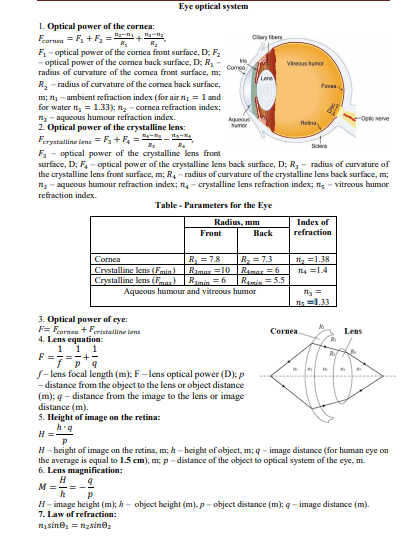help_outlineImage TranscriptioncloseEye optical system 1. Optical power of the cornea: Fornea = F, + F = F- optical power of the cornea front surface, D; F - optical power of the cornea back surface, D; R, radius of curvature of the comea front surface, m; Clary fibers R. Vous humor Comea Lene - radius of curvature of the comea back surface, R, Fora m; n1 - ambient refraction index (for air n = 1 and for water n, = 1.33); n - cornea refraction index: n- aqueous humour refraction index. 2. Optical power of the erystalline lens: Ferystalline tens =F +F, = s-a E- optical power of the crystalline lens front surface, D; F, - optical power of the crystalline lens back surface, D; R - radius of curvature of the crystalline lens front surface, m; R, - radius of curvature of the crystalline lens back surface, m; n - aqueous humour refraction index; n, - crystalline lens refraction index; n; - vitreous humor Oplo nerve Agueous humor Rena Scera refraction index. Table - Parameters for the Eye Radius, mm Index of refraction Front Back R = 7.8 Ramar =10 Ramin =6 Aqueous humour and vitreous humor R, = 7.3 R4maz =6 Ramie =5.5 Cornea n, =1.38 n4 =1.4 Crystalline lens (Fmin) Crystalline lens (Fmar) =1.33 3. Optical power of eye: F= Farnaa + Feristalline lens 4. Lens equation: 1 1. 1 Cornea Lens S- lens focal length (m); F- lens optical power (D); p - distance from the object to the lens or object distance (m); g – distance from the image to the lens or image distance (m). 5. Height of image on the retina: h.9 Н Е H - height of image on the retina, m; h - height of object, m; q - image distance (for human eye on the average is equal to 1.5 cm), m; p - distance of the object to optical system of the eye, m. 6. Lens magnification: н H- image height (m); h - object height (m), p - object distance (m); g - image distance (m). 7. Law of refraction: nisine, = nzsinez fullscreen
check_circle

Focusing power of crystalline lens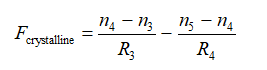(a) Minimum focusing power of crystalline lens

When focused at distant object the radius if curvature of the crystalline lens is maximum.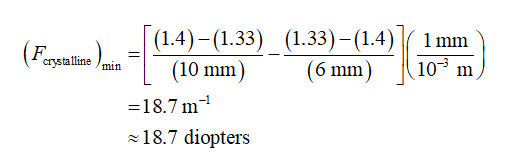(b) Minimum focusing power of the eye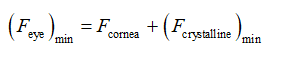...

### Want to see the full answer?

See Solution

#### Want to see this answer and more?

Solutions are written by subject experts who are available 24/7. Questions are typically answered within 1 hour.*

See Solution
*Response times may vary by subject and question.
Tagged in

### Physics